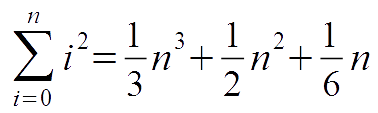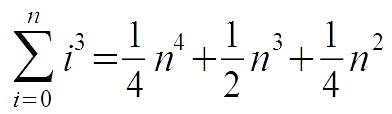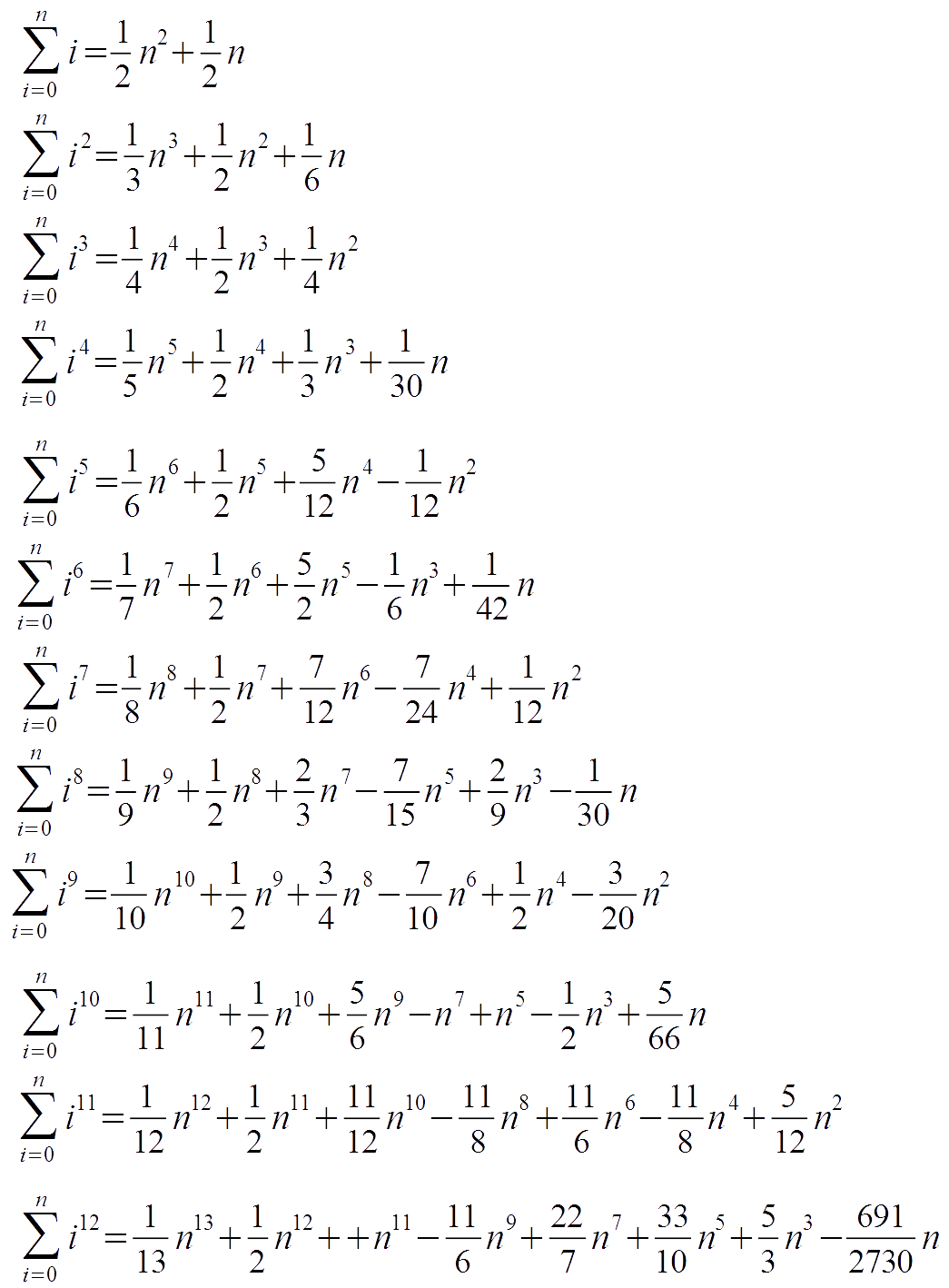# Sum of the First Quadratic Numbers

or The Pyramidal Number

## Introduction

What is the sum of the first n quadratic numbers? Fascinated from the easy way of calculating the sum of the first natural numbers, I studied if there was an equal nice way of doing it for the quadratic numbers. At the time when I studied the subject I was not able to find an equally easy and nice solution as Carl Friedrich Gauß found it for the natural numbers, however I found a logic and easy to follow approach which works also for sums of higher order, and which is mathematically correct.=## What is the problem?

What is the result of the following expression: 12 + 22 + 32 + 42 + 52
Well, just calculate it and you know: 1 + 4 + 9 + 16 + 25 = 55

OK, this was easy, but know what is the result of this expression: 12 + 22 + 32 + 42 + 5 + ... + n2
Well, one could calculate it with a simple computer program, or one thinks about it and might find the solution which works for all n and which is calculated quicker than by the simple computer program ... which is what this article is about.

The mathematical question is the following:## What is the solution?

The formula for the problem is:## Find the solution? The quick way.

Looking nowadays through the Internet shows that many people considered the same problem. Interesting is that there are many different methods to come to the conclusion. A nice way is described on the following link: http://www.arndt-bruenner.de/mathe/Allgemein/summenformel1.htm

The approach I took started similar as in the above link, however I found another very obvious fact. Having assumed that there is an existing formula for the sum, the slope of that function must be quadratic so the function we look for must be of 3rd power. So we know that the formula is of this structure:With 4 equations this problem is solved (e.g. set n=0, we find out that d=0 - with n=1, n=2, and n=3 we get a linear system of equations which result in a=1/3, b=1/2, and c=1/6).

## The next step

Using the same logic and method we can find answers to sums of numbers of higher power.

Sum of cubic numbers:List of sums of higher order: